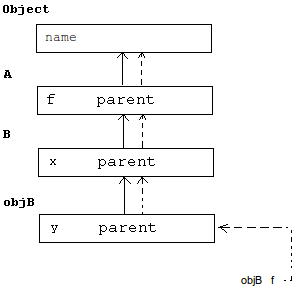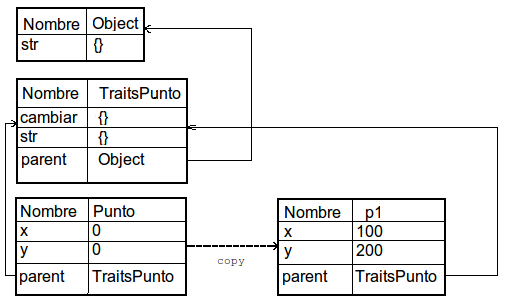# POOI - An object-oriented, protoype-based programming system interpreter.

## Introduction

POOI is an object-oriented prototype-based programming system interpreter, designed with education in mind. It supports its own programming language, which was designed to be very simple. Objects send message among them in order to get a program that solves a problem. Everything is an object, including literal data.

``` <object> <message> [ <arg1> [ <arg2> [...] ] ] ```

For instance, in order to see all members (attributes (i.e., data) and methods (i.e., code answering messages), it is possible to just write a message as follows: Object list. This will display the information in the output window. Object is the root of the inheritance hierarchy, in which the messages supported by all objects (such as str or list) reside). As another example, 5 str would convert the integer literal 5 into a string.

• list - Returns a textual representation of all members in the object.
• str - Returns a textual representation of the object.
The main difference is that the second, if not overwritten, will just include the values of the attributes. The first one includes a detailed description for all members.

## Basic interpreter workings

POOI is able to process data of the following kinds:
• Bool - Logic values: true or false.
• Int - 64bit integer numbers.
• Real - 64bit real numbers.
• Str - Limitless strings.
It is possible to easily do mathematical calculations, such as the following ones (the symbol > identifies inputs, in contrast to the interpreter's answers):

``` > 5 + 6 11 > 6 * 5 30 ```

In order to get the list of messages supported by integer numbers, you can type:

` Int list `

The same method is valid for real numbers:

` Real list `

...and for strings:

` Str list `

You can write complex messages by grouping or nesting them by means of brackets.

` (5 * 6) + 2 `

Firstly the multiplication of 5 and 6 is calculated, and the result is added to 2. Messages are interpreted always from left to right, without need of any kind precedence, since the brackets impose a strict sequence.

` (5 * 6) + (4 / 2) `

This code will produce the same result as the same one.

What is really happening is that the interpreter assumes the first term as the destintation of the message, while the message appears then with its arguments following. The message Int list message does not have arguments, it does only have a message list being sent to the object Int. In a slightly more complex message, such as 5 * 2, the object 5 (an integer literal) receives the multiplication message, with 2 as its first an only argument.

### Creating objects

New objects are created by copying them. For instance, a message to create a completely new object could be:

```> anObject copy anObject was copied as anObject4 ```

This will make a copy of anObject. Since anObject is empty, the new object will also be empty. If you want to give it a new name, it is possible by means of the message rename, which accepts a new name (as a string) as its parameter.

```> anObject4 rename "obj" anObject4 was renamed as obj ```

As it has been shown above, it is possible to combine both messages in a single, more complex, command:

```> (anObject copy) rename "obj" anObject was copied as anObject4 anObject4 was renamed as obj```

In order to confirm which members are present in the new object, it is possible to use both list and str. As discussed above, they give a textual representation of the object, with different level of detail.

```> obj str { } > obj list Object obj = {     parent = Object } ```

### Adding members to an object

For the object to be useful, it should contain data and be able to execute actions over it. The former is achieved with attributes, while the latter can be achieved with methods.

```> (anObject copy) rename "Point" anObject was copied as anObject4 anObject4 was renamed as Point```

In this example, a cartesian point with two coordinates will be created, x and y. Adding both new coordinates to the Point is as simple as shown below:

`> Point.x = 0`
`> Point.y = 0 `

If the message str is sent, then we will be able to verify that it now has two attributes, two integer numbers with value zero.

```> Point str { x = 0 y = 0 }```

The message set is equivalent to Point.x = 0, in the form of Punto set "x" 0. It adds a new attribute to the object if it does not exist, or modifies its values if it does exist. The very same object, with the changed applied is returned, so it is possible to chain various assingments in just one command. Therefore the notation "=" is just syntactic sugar.

New behaviour can be easily added to the object by creating methods that will do the needed tasks. For example, it could be needed to have a method to change the location of the point, i.e., changing the value of x and y. This method could be called moveTo, sporting two parameters.

```> Point.moveTo = {a b: self.x = a; self.y = b} 'moveTo' set in Root.Punto ```

Methods are delimited with braces: { and }. Following the left brace, a list of formal parameters follows, separated from the body of the method with a colon (':'). This parameters will hold the real arguments sent with the message. Inside a method, it is possible to send the same kind of messages that have been discussed until now. When it is needed to reference the object receiving the message, the self prefix must be used. With all of this in mind, the code above means that the values for the attributes x and y will be the values passed as real arguments with the moveTo message.

```> Point moveTo 10 20 'x' set in Root.Point 'y' set in Root.Point > Point str { x = 10 y = 20 }```

A more elaborated output than the one provided by str by default would be interesting. Both coordinates separated by a comma, could be more intuitive:

```> Point.str = {: ( ( self.x str ) + ", " ) + ( self.y str ) } 'str' set in Point > Point str 10, 20 ```

In the code above the method str in Point has been executed instead the default one, present in Object. This is an interesting oportunity to discuss more deeply the model of orientation supported by Pooi.

## The prototype-based model

Typical object-based programming languages, such as C++ or Java, are class-based. Classes are like blueprints for new objects, so the structure of objects pertaining to a given class will be always the same, they will only differ in the values of the fields. The inheritance mechanism provided in these systems is inheritance by concatenation, so an object is the result of the concatenation of the attributes of all superclasses of its class, plus the ones present in his class.

The object-oriented model supported by Pooi does not have classes. Objects are simply copied from each other, and and its members can be erased, while new members can be added. Objects representing the same concept do not mandatorily have to be structurally identical. Some objects are used as a reference instance from which new objects will be copied. This objects are called prototypes, reforcing the fact that those new objects can be similar to them, but not necessarily identical. Since classes do not exist, inheritance by concatenation does not exist either, the available mechanism is inheritance by delegation.In the figure above, it is possible to see the route needed to execute message f. Object objB reveives a message f. However, objB does not containt the member f, so it delegates the execution of the method in its parent object. An object knows its parent through an attribute called parent that always exist (an object cannot be really empty) and points to it. Object B does not have a member called f either, so the parent attribute is followed in order to reach object A. This object do have an f member, so the method f is executed inside object A, with objB as target or objet which is executing the method (pointed by the reference self).

```> Point list Object Point = {     str = {: ( self.x str ); ( __POP + ,  ); ( self.y str ); ( __POP + __POP );  }     moveTo = {a b: ( self set x a ); ( __POP set y b );  }     x: Int = 10     parent = Object     y: Int = 20 } ```

In this example, an object Point, which will be used as a prototype, contains two methods an two attributes. In order to create a new instance of Point, you will have to copy it.

```> (Point copy) rename "p1" ````Point```` was copied as anObject5 ````anObject5`` was renamed as p1`

Now, it is possible to use p1 as a Point, and leave Point reserved to create new future objects Point.

```> p1 moveTo 11 12 ``````x set to 11 y set to 12 > p1 str 11, 12 ```

However, in order to create p1 both methods moveTo and str had to be copied as well. It probably does not make sense to copy two methods that will be identical in all Point objects. This would result in both a performance and memory waste problem. In the class-based model this problem does not exist, since methods are never copied.

A feasible alternative is to divide the prototype in state and behaviour (TraitsPoint). These two objects, Point and TraitsPoint will be related by inheritance. The former will held the state, while the latter will contain all needed methods (behaviour).

```> (anObject copy) rename "TraitsPoint" 'anObject6' renamed as 'TraitsPoint' > ````TraitsPunto````.moveTo = {a b: self.x = a; self.y = b} 'moveTo' set in Root.Punto ``````> (anObject copy) rename "Point" ``````'anObject7' renamed as 'Point' > Point.x = 0```
```> Point.y = 0 ``````> Point.parent =  TraitsPunto ``````> (Point copy) rename "p1" 'Point4' renamed as 'p1' > p1 moveTo 100 200 > p1 str 100, 200 ```

The resulting schema is shown below.Due to this, it is said that the prototype-based model is able to represent the class-bed model.